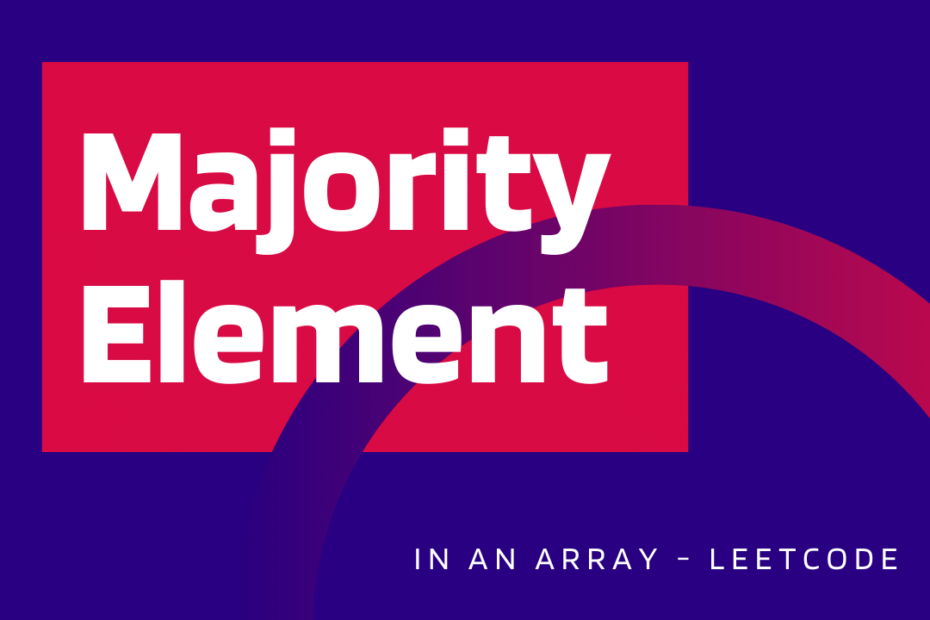# Majority Element in an Array – LeetCode

Given an array of size n, find the majority element. The element that appears more than ⌊ n/2 ⌋ times in the array. Note: Input will be a non-empty and unsorted array.

Example

``````Input: [2,1,2]
Output: 2

Input: [1,1,2,2,2,1,1]
Output: 1``````

## 1. Sorting

On sorting the given array, the element occurring more than [n/2] times will be present at the middle of the array. But, sorting an array takes O(nlog(n)). So the time complexity of this approach becomes nlog(n).

``````#include <algorithm>
class Solution
{
public:
int majorityElement(vector<int> &nums)
{
sort(nums.begin(), nums.end());
return nums[nums.size() / 2];
}
};``````

` Runtime: 72 ms Memory: 20.1 MB `

Time Complexity:O(nlog(n))
Space Complexity:O(1)

## 2. Using Hash Map

We can use a Hash Map to iterate through the array for once and then return the array that occurs more than [n/2] times. As we are iterating only once the Time complexity would be O(n) and the hashmap does take extra space of O(n).

``````class Solution
{
public:
int majorityElement(vector<int> &nums)
{
unordered_map<int, int> map;
int n = nums.size();
for (int i = 0; i < n; i++)
{
map[nums[i]]++;
if (map[nums[i]] > n / 2)
return nums[i];
}
return -1;
}
};``````

` Runtime: 48 ms Memory: 20.1 MB `
Time Complexity:O(n)
Space Complexity:O(n)

## 3. Without using Hash Map

Hash Map does take a lot of space but, in this method we cancel out the occurrence of each non-majority element to the majority element. Say, there are 7 elements, there would be 4 majority elements and 3 non-majority elements.

``````class Solution
{
public:
int majorityElement(vector<int> &nums)
{
int count = 0, major;
for (auto a : nums)
{
if (count == 0)
{
major = a;
count = 1;
}
else if (major == a)
{
count++;
}
else
{
count--;
}
}
return major;
}
};``````

` Runtime: 32 ms Memory: 20 MB `

## Complexity for majority element

Time Complexity:O(n)
Space Complexity:O(1), no extra space.